New Students Offer - Use Code HELLO

# Time Speed And Distance Tricks: Part 1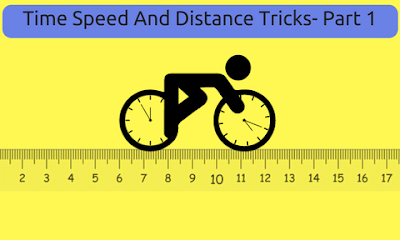1. Basic Formulas:Example: If a person travels some distance in 2 hours at a speed of 20 km/h.
Solution:

D=S×T
D= 20×2
D = 40 km.

2. To convert from Km/h to m/s multiply by 5/18. Example: A person travels 72 km distance in 4 hours. Find his speed in m/s.
Solution:

3. To convert from m/s to Km/h multiply by 18/5.
Example: A person crosses a 900 m long street in 10 minutes. Find his speed in Km/h.
Solution:4. If a person travels some distance at x km/h instead of y km/h, he would have walked ‘a’ km more, then actual Distance:Example: If Mohan walks at 18 km/h instead of 12 km/h he can cover 48 km more. What is the actual distance?
Solution:5. If a person travel some distance at x km/h instead of y km/h, he would have walked ‘a’ km less, then actual Distance:Example: If Sushil walks at 40 km/h instead of 45 km/h he can cover 10 km less. What is the actual distance?
Solution:6. If a person travels some distance travels hours. He covers half of the distance at x km/h and second half speed at y km/h then Distance: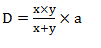Example:  If a man covers certain distance in 12 hours by scooter. He covers half of the distance at a speed of 25 km/h and another half distance at a speed of 15 km/h. What will be the distance covered by him?
Solution:7. A person takes x hour in walking to a certain distance and riding back. If he takes y hour by riding both sides then time taken by both side by walking: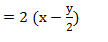Example: Sushant takes 7 hour 40 minutes in walking to a certain distance and riding back by car. If he takes 5 hour 20 minutes by walking both sides then find time taken by riding both sides.
Solution:8. If a person covers some distance at a speed of x km/h, he gets late by ‘a’ hour. If he covers same distance at a speed of y km/h, he reaches ‘b’ hour early then distance: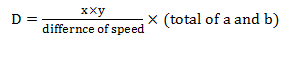Example:  If Seema covers ‘A’ distance at a speed of 60 km/h, he gets late by 20 minutes. If she covers same distance at a speed of 55 km/h, she reaches 10 minute early. What is value of A?
Solution: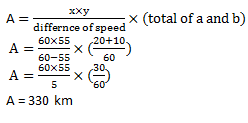9. If a person covers some distance at a speed of x km/h, he gets late by ‘a’ hour. If he covers same distance at a speed of y km/h, he gets late by ‘b’ hour, then distance: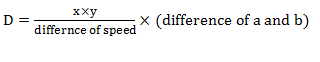Example:  If Rohan covers ‘A’ distance at a speed of 36 km/h, he gets late by 10 minutes. If he covers same distance at a speed of 40 km/h, she gets 6 minute late. What is value of A?
Solution:10. If a person covers some distance at a speed of x km/h, he reaches early by ‘a’ hour. If he covers same distance at a speed of y km/h, he reaches early by ‘b’ hour, then distance: